# R plot pch symbols – Different point shapes available in R

• Last Updated : 03 Dec, 2021

In this article, we will discuss Pch that are built-in shapes in the R programming language.

The pch (plot characters) in the R programming language are symbols or shapes we use for making plots. In the R Language, there are 26 built-in shapes that can be used in any graphic work in R Language and can be identified by numbers ranging from 0 to 25. The first 19 symbols are also known as s-compatible vector symbols and the remaining 7 are called the R-specific vector symbols. Characters other than numbers can also be used to specify pch including “+”, “*“,”-“,”.“,”#, “%”, “o”.

When we make a basic scatter plot, by default, it uses a dot to represent data that is pch=16. But we can convert it to any other shape using the desired pch value. Since remembering the pch value for each shape is difficult we can create a plot like the following to get the values of each symbol.

Example: Displaying all pch symbol

## R

 `# load library tidyverse``library``(tidyverse)` `# create dataframe of 26 character to``# visualize the 26 characters``df <- ``data.frame``(p=``c``(0:25)) %>%``            ``mutate``(x = ``rep``(``seq_len``(``ceiling``(``n``()/6)),``                  ``each = 6,``                  ``length.out = ``n``())) %>%``                  ``group_by``(x)%>%``                  ``mutate``(y=1:``n``())` `# create plot using ggplot function``# shape parameter is used to shape points``ggplot``(df, ``aes``(x = x, y = y, shape = p),) +``  ``scale_shape_identity``() +``  ``geom_point``( size = 6, fill = ``"green"``)+` `# geom_text function is used to label data points``# with pch value``geom_text``(``aes``(x = x - 0.3, y = y,``                ``label = ``paste0``(``"pch ="``,p)), size = 3)`

Output: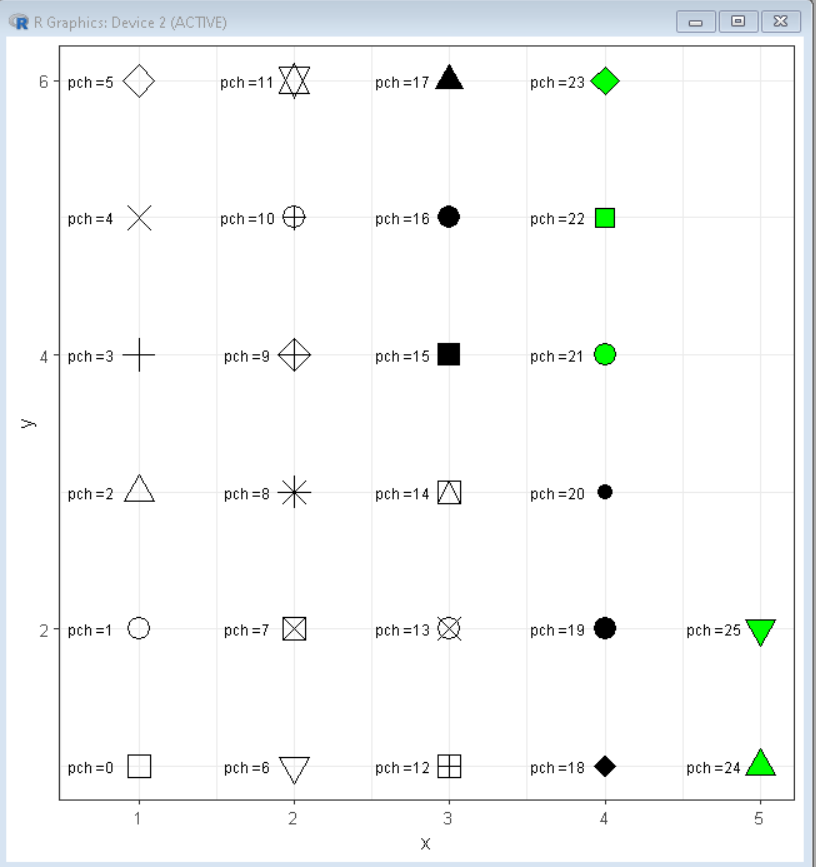Following are the Pch value for different shapes.

## Using special characters as Pch values

Some special characters can also be used as values of the pch symbol instead of the numbers. In the R Language, we can use “+”, “*“,”-“,”.“,”#, “%”, and “o” for plotting different symbols. These symbols convert the pch shape exactly to their own shape. Below is a plot showing these symbols in action.

Example: Using special symbols as Pch values

## R

 `# create sample data frame``xAxis <- ``rnorm``(100)                ``yAxis <- ``rnorm``(100) + xAxis + 10 ` `# create plot``# use pch function to shape plot points``# using pch value``plot``(xAxis, yAxis, pch = ``"%"``, size=9)`

Output: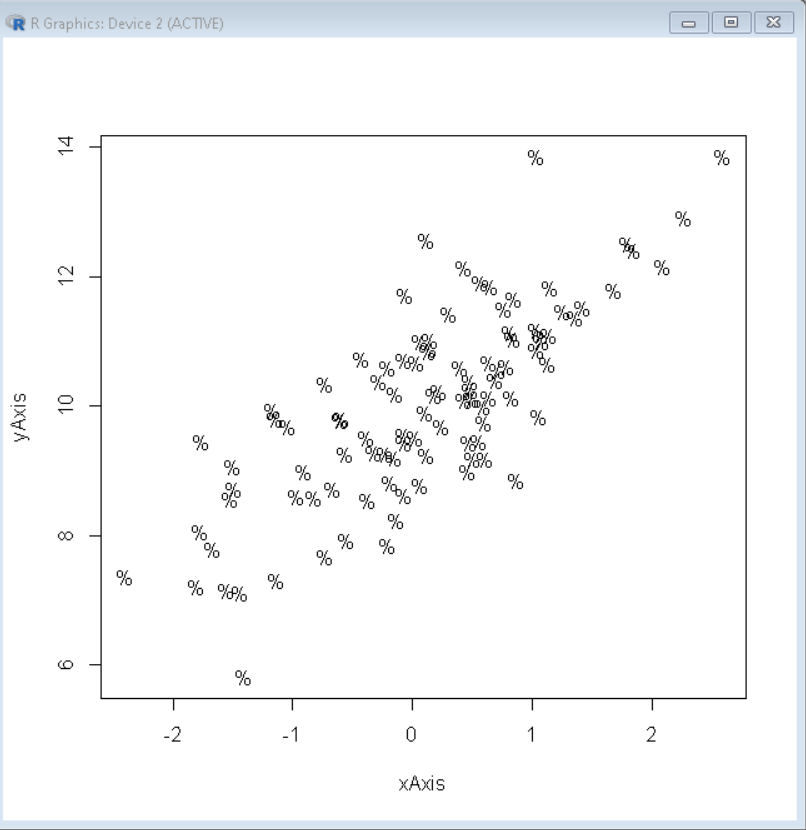## Using shapes in ggplot plot

Now we can plot, scatter plot using the desired shape by putting the pch number at a shape value. We can use these shapes at any place in the R Language where shape vectors are used.

Example: A basic scatter plot with star shape using pch value as 8

## R

 `# create sample data frame``xAxis <- ``rnorm``(100)                ``yAxis <- ``rnorm``(100) + xAxis + 10 ``df <- ``data.frame``(xAxis, yAxis)` `# load library ggplot2``library``(ggplot2)` `# create plot``# use shape argument to shape plot``# points using pch value``ggplot``(df,``aes``(x=xAxis, y=yAxis))+``geom_point``(shape = 8)`

Output: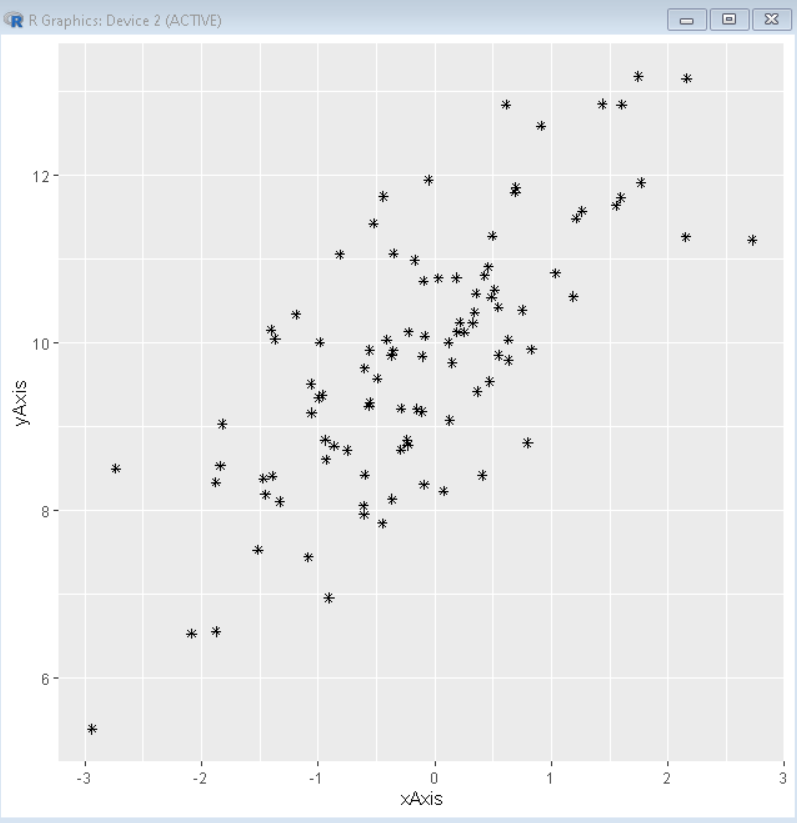## Omitting points from the plot

To omit or remove points from any plot, we can use the pch value of NA. This makes the shape of points in a plot as zero-sized or invisible that giving an impression of removal of points.

Syntax:

plot( x, y , pch= NA )

Example: Removing all points

## R

 `# create sample data frame``xAxis <- ``rnorm``(100)                ``yAxis <- ``rnorm``(100) + xAxis + 10 ``df <- ``data.frame``(xAxis, yAxis)` `# load library ggplot2 and gridExtra``library``(ggplot2)``library``(gridExtra)` `# create plot``# use pch function to shape plot points``# using pch value``plot1 <-``ggplot``(df,``aes``(x=xAxis, y=yAxis))+``        ``geom_point``(shape = ``"*"``, size=10)` `plot2 <-``ggplot``(df,``aes``(x=xAxis, y=yAxis))+``        ``geom_point``(shape = ``NA``)` `# combine plots using grid.arrange() function``grid.arrange``(plot1, plot2, ncol=2)`

Output: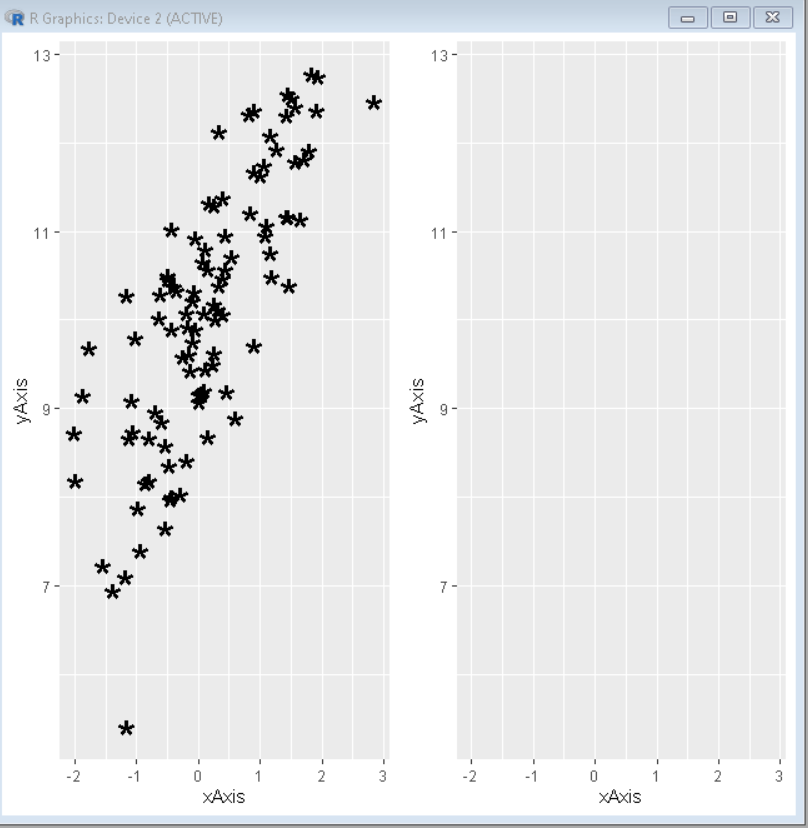## Color Customization

We can change the color of the outline, background fill, size of the symbol, and line width of the outline by using col, bg, cex, and lwd parameters respectively.

Syntax:

plot( x, y, pch, cex, bg, col, lwd )

Parameter:

• col : determines the color for the outline of the shape
• bg : determines the color for the background fill of the shape
• cex : determines the size of the pch symbol
• lwd : determines the line width for the the pch symbol outline

Example: Adding color customization to pch symbols

## R

 `# create sample data frame``xAxis <- ``rnorm``(100)                ``yAxis <- ``rnorm``(100) + xAxis + 10 ` `# create plot``# use pch function to shape plot points``# using pch value``plot``(xAxis, yAxis, pch = 21, cex=3, col=``"green"``,``     ``bg=``"yellow"``, lwd=4)`

Output: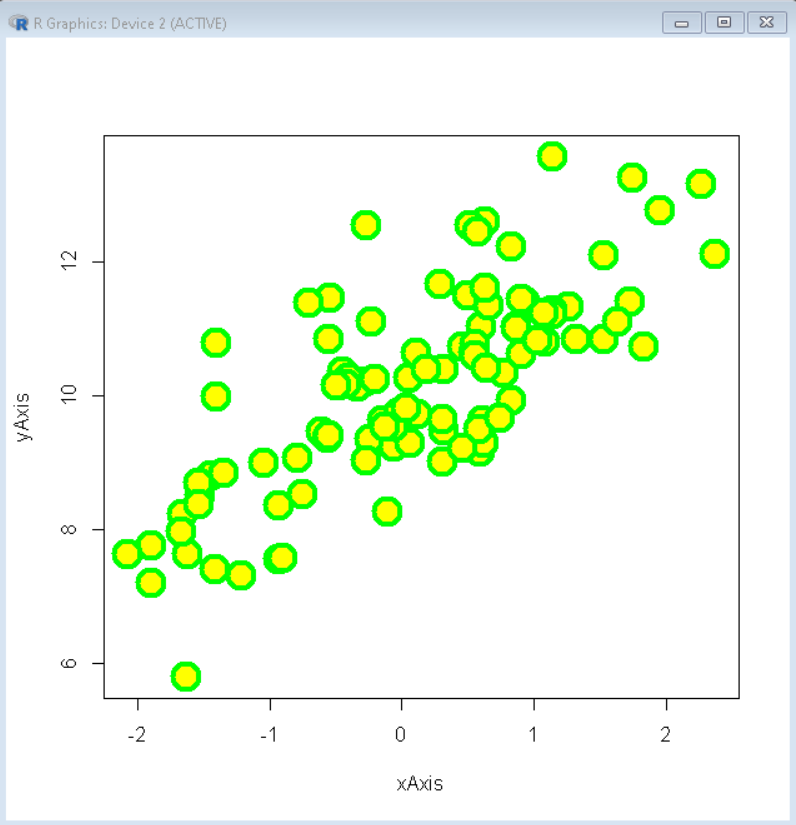My Personal Notes arrow_drop_up